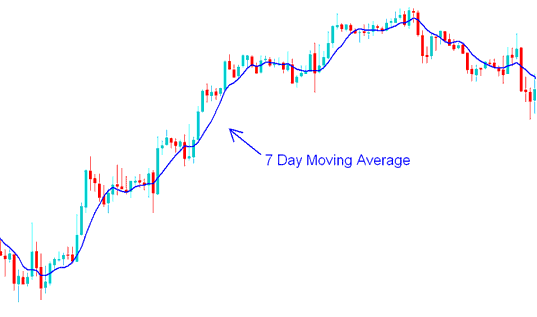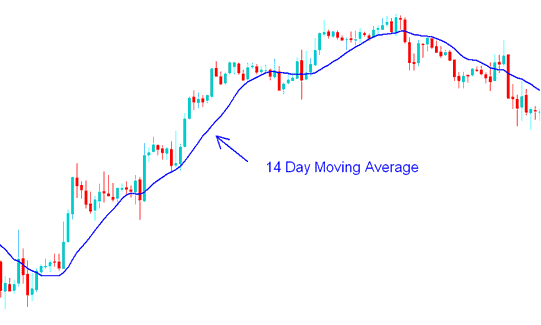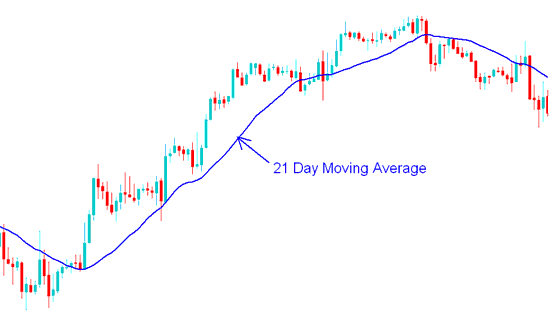# Trading Short-Term and Long-Term XAUUSD Price Period of Moving Average

A xauusd trader can choose to adjust the gold price periods used to calculate the moving average.

If a xauusd trader uses short gold price periods then the MA will react faster to the changes in xauusd price.

For example if a xauusd trader uses the 7 day xauusd moving average then, the moving average indicator will react to the gold price change much faster than a 14 day or 21 day xauusd Moving Average would. However, using short time gold price periods to calculate the MA might result in the indicator giving false xauusd signals (whipsaws).7 Day Moving Average - Moving Average XAUUSD Strategies

If another trader uses longer time periods then the MA will react to gold price changes much slower.

For example, if a xauusd trader uses the 14 day MA then the average will be less prone to whipsaws but it will react much slower.14 Day Moving Average - Moving Average XAUUSD Strategy Example21 Day Moving Average - Moving Average XAUUSD Strategies Example